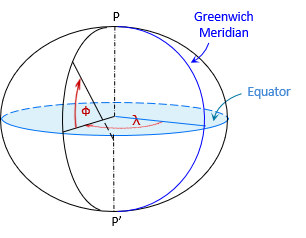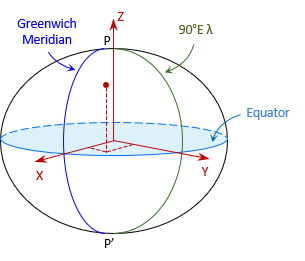### 3. Coordinate System

Part of datum definition is creation of a coordinate system for the entire mathematical model. Its origin is set at, or very close to, the Earth's mass center.

#### a. Geodetic Coordinates

Geodetic coordinates are similar to geographic coordinates, except they are based on an ellipsoid instead of a sphere.

The sphere on which geographic coordinates are based, Figure C-7, has a constant radius throughout.Figure C-7 Geographic Coordinates

The line from the observer's position is radial to the sphere center and normal to the sphere's surface, Figure C-8.Figure C-8 Geographic Latitude

The geographic latitude is the angle in the observer's meridian from the equator to the radial line.

With an ellipsoid, the line normal to its surface at the observer's position does not extend to the ellipsoid center, Figure C-9, except at the poles and equator.Figure C-9 Geodetic Latitude

The normal terminates on the minor axis. The geodetic latitude, φ,  is the angle from the major axis to the normal line.
The geodetic longitude, λ, is the angle in the equatorial plane between the Greenwich and observer's meridians.
Geodetic latitude and longitude are shown in Figure C-10.Figure C-10 Geodetic Coordinates

A point's 3D position is given by its geodetic coordinates and ellipsoidal height.

#### b. Linear Coordinates

Geodetic coordinate have similar disadvantages as geographic coordinates. An alternative is the Terrestrial Reference System (TRS), a 3D orthogonal linear coordinate system, Figure C-11.Figure C-11 Terrestrial Reference System

Like geodetic coordinate system, it is a geocentric system. The X and Y axes are in the equatorial plane with X passing through the Greenwich Meridian and Y through 90°E longitude. The Z axis is perpendicular to the equatorial plane and positive through the North Pole.

A point's 3D position is given by its (X,Y,Z) coordinates.

Although TRS is a linear system, it is not convenient for surveying or mapping applications because of coordinate magnitudes. For example, the coordinates of a control point in southcentral Wisconsin is  21,919.631m X, -4,679,204.603m Y, 4,320,134.562m Z. Their size makes them cumbersome to work with.